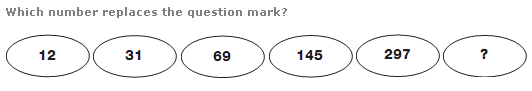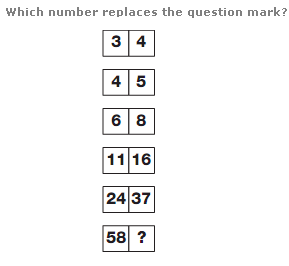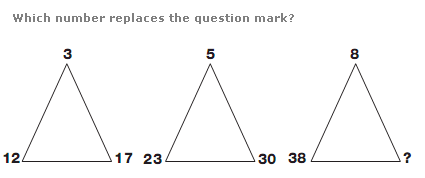# Puzzles - Number puzzles

### Exercise :: Number puzzlesAnswer : 601 Explanation : Starting at the top, double each number and add 7 to give the next number down.Answer : 92 Explanation : Working from top to bottom, left to right, add together the first two numbers and subtract 3 to give the next value along.Answer : 47 Explanation : Starting at the top on the left, and working in rows across the 3 triangles, values increase by 2, then 3, then 4 etc.